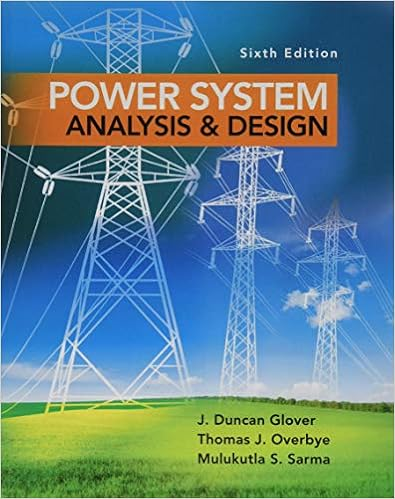Lab 1.docx - EENG 3224/6633 Digital Signal Processing Lab...

• 2

This preview shows page 1 - 2 out of 2 pages.

We have textbook solutions for you!
The document you are viewing contains questions related to this textbook.The document you are viewing contains questions related to this textbook.
Chapter 5 / Exercise 5.34
Power System Analysis and Design
Glover/OverbyeExpert Verified
EENG 3224/6633 Digital Signal Processing Spring 2019 Lab 1 Prof. H. Silver
We have textbook solutions for you!
The document you are viewing contains questions related to this textbook.The document you are viewing contains questions related to this textbook.
Chapter 5 / Exercise 5.34
Power System Analysis and Design
Glover/OverbyeExpert Verified
The MATLAB program below generates and attempts to recover a sampled 1 Hz. sine wave, using the Fast Fourier Transform (fft) and Inverse Fast Fourier Transform (ifft) functions. The fft, to be discussed in the future, results in a spectrum of N-1 complex coefficients. The coefficients above N/2 are complex conjugates of corresponding lower coefficients. The program plots the magnitudes of the coefficients, relative to Xn(1). (a) Create and save the program. You can omit the comments. Run the program and input 64 samples for N. Caution: Note the use of both lower case x in the time domain and upper case X in the frequency domain.
•••